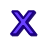Games
Problems
Go Pro!

# Multiplication

Quizzes > Mathematics > Arithmetic > Multiplication

# Featured QuizzesPractice your multiplication tables with this quiz. Students who remember their multiplication facts work much more efficiently than students who...Practice your multiplication facts with this simple math quiz at The Problem Site.Practice your math facts with this simple math quiz at The Problem Site.Practice your multiplication facts with this simple math quiz at The Problem Site.Practice your multiplication facts with this simple math quiz at The Problem Site.Practice your multiplication facts with this simple math quiz at The Problem Site.Practice your multiplication facts with this simple math quiz at The Problem Site.Practice your multiplication facts with this simple math quiz at The Problem Site.

# Full Directory Listing

Multiplication by 2, Multiplication by 3, Multiplication by 4, Multiplication by 5, Multiplication by 6, Multiplication by 7, Multiplication by 8, Multiplication by 9, Multiplication Facts (All)Like us on Facebook to get updates about new resources